#### the "encoding" of the distribution of prime numbers by the nontrivial zeros of the Riemann zeta function [common approach]

The distribution of prime numbers is most simply expressed as the (discontinuous) step function, whereis the number of primes less than or equal to x.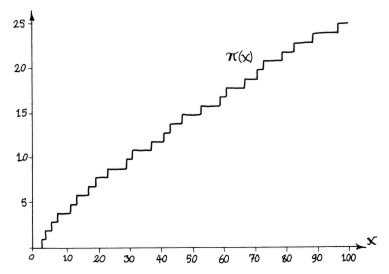It turns out thatcan be expressed exactly as the limit of a sequence of smooth functions Rn (x). To define Rn(x) we first introduce the logarithmic integral function Li(x), which appears throughout the analytical theory of the prime distribution:This is a smooth function which simply gives the area under the curve of the function 1/log u in the interval [2,x].

Don Zagier explains the reasoning behind the function Li in his excellent introductory article "The first 50 million prime numbers" (from The Mathematical Intelligencer 0 (1977) 7-19, based on his inaugural lecture held at Bonn University, May 5, 1975):

"[A] good approximation to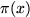, which was first given by Gauss is obtained by taking as starting point the empirical fact that the frequency of prime numbers near a very large number x is almost exactly 1/log x. From this, the number of prime numbers up to x should be approximately given by the logarithmic sum

Ls(x) = 1/log 2 + 1/log 3 + ... + 1/log x

or, what is essentially the same, by the logarithmic integralUsing Li(x) we then define another smooth function, R(x), first introduced by Riemann in his original eight-page paper, and given by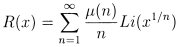Here the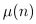are Möbius Numbers. Certain useful comments and graphs relating to this function appear in Riesel's book.

In the aforementioned article, Don Zagier goes on to explain the reasoning behind the Riemann function:

"There is one more approximation which I would like to mention. Riemann's research on prime numbers suggests that the probability for a large number x to be prime should be even closer to 1/log x if one counted not only the prime numbers but also the powers of primes, counting the square of a prime as half a prime, the cube of a prime as a third, etc. This leads to the approximation: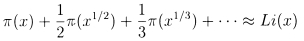or, equivalently [by means of the Möbius inversion formula]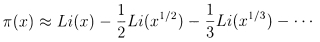[Note that the minus signs result from Möbius numbers and do not continue indefinitely]

The function on the right side of this formula is denoted by R(x), in honour of Riemann. It represents an amazingly good approximation to...

For those in the audience who know a little function theory, perhaps I might add that R(x) is an entire function of log x, given by the rapidly converging power series: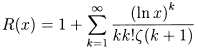where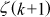is the Riemann zeta function."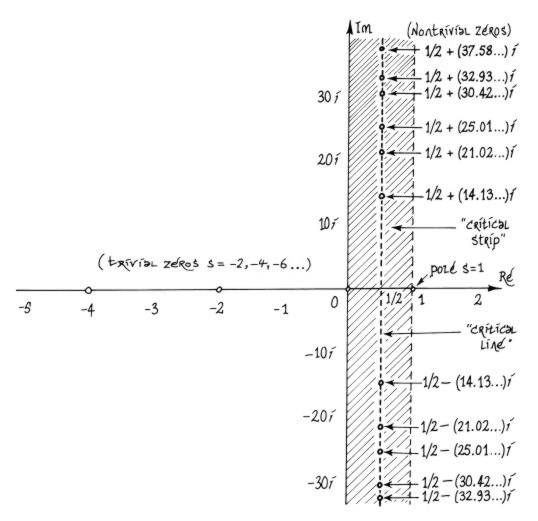Here we see the zeros of the Riemann zeta function in the complex plane. These fall into two categories, trivial and nontrivial zeros.

Here are some tables on nontrivial zeros compliled by Andrew Odlyzko.

The trivial zeros are simply the negative even integers. The nontrivial zeros are known to all lie in the critical strip 0 < Re[s] < 1, and always come in complex conjugate pairs. All known nontrivial zeros lie on the critical line Re[s] = 1/2. The Riemann Hypothesis states that they all lie on this line.

The difference between the prime counting function and its "amazingly good approximation" R(x), i.e. the fluctuations in the distribution of primes, can be expressed in terms of the entire set of zeros of zeta, which we shall represent by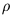, via the function R itself: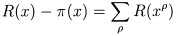Obviously some of the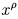are complex values, so here R is the analytic continuation of the real-valued function R defined previously. This was mentioned above by Zagier, and is known as the Gram Series expansion:The sum overseparates into two sums, over the trivial and nontrivial zeros, respectively. The former sum is of course just R(x-2) + R(x-4) + R(x-6) + ..., and the latter can be written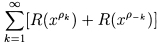The contributions from the complex-conjugate pairs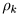and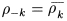cancel each others' imaginary parts, so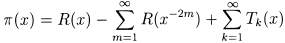where theare real-valued.

We can now define the sequence of functions Rn(x) which approximatein limit: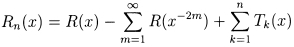An excellent illustration of this is provided on page 55 of Hans Riesel's book, which compareswith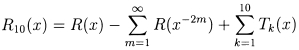i.e. the Riemann function corrected by contributions from the first 10 pairs of nontrivial zeta zeros. Zagier's article also includes graphs of the first few Tk(x) as well as R10(x) and R29(x).

Below we see an animated image, each successive frame being the result of adding another Tn(x) to the function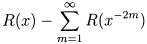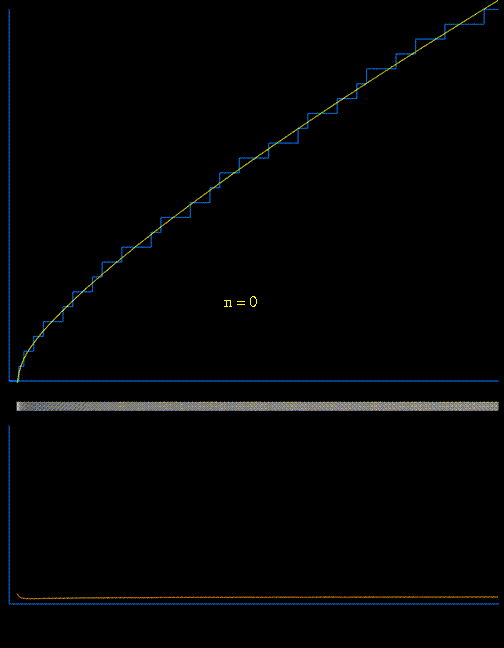[animation courtesy of Raymond Manzoni]

The lower animated graph is the derivative of the function above, and we see the positions of the primes emerging as Dirac delta-type spikes. The horizontal bar in the middle has been constructed so that the intensity of brightness is related to the absolute value of the derivative. In this way we see the positions of the primes gradually emerge as narrow bands of light.

To summarise: R(x) is asymptotic to, and is a particularly good estimate for the distribution of primes. It is a refinement of Li(x), itself a refinement of the basic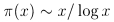given by the prime number theorem. R can be thought of as describing the "average" behaviour of the primes. The correction terms Tk(x), which are defined using the zeros of the zeta function collectively describe the local fluctuations.

It is quite remarkable that a single smooth function can provide not only an excellent estimate for, but also, through an infinite sum of (complex) exponential "rescalings", an exact expression for the fluctuations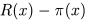.

In other words, with the single function R and the set of nontrivial zeta zeros, we can exactly reconstruct the prime counting function. Consequently, the sequence of nontrivial zeta zeros is sometimes described as being "dual" to the sequence of primes.

the more elegant approach

### further items of interest

"We will...write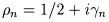...Nothing is known about the, but they are thought likely to be transcendental numbers, algebraically independent of any reasonable numbers that have ever been considered."

A.M. Odlyzko on the nontrivial zeros of the zeta function, from "Primes, Quantum Chaos, and Computers"

"What Riemann observed (and Hadamard later proved) was that the zeta function can also be written as a product over its zeroes in the complex plane: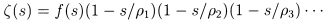where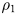,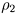, etc. are the complex numbers for which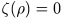, and f(s) is a fairly simple "fudge factor". Riemann showed that by equating the logarithms of these two expressions for the zeta function it's possible to derive...a whole sequence of increasingly accurate approximations - in effect, an exact formula for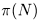."

Barry Cipra, from "A Prime Case of Chaos" (1999)

"One of Riemann's great achievements [1,2] was to give an exact formula for, constructed as follow. First,is expressed in terms of a function J(x) [1, Chapter 1] that has jumps at prime powers: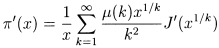In this formula,are the Möbius numbers (1, –1, –1, 0, –1, 1, ...). Each of the partial densities J ' is the sum of a smooth part and an infinite series of oscillations:(see section 1.18 of ). Here the numbers tn in the oscillatory contributions are related to the complex Riemann zeros...It is known that the complex zeros (i.e., those with nonzero imaginary part) of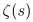lie in the "critical strip" (Re(s) between 0 and 1) , and the Riemann hypothesis states that in fact all these zeros lie on the "critical line" Re(s) = 1/2. The numbers tn are defined byIf the Riemann hypothesis is true, all the (infinitely many) tn are real, and are the heights of the zeros above the real s axis. It is known by computation that 1,500,000,001 complex zeros lie on the line ."

M.V. Berry and J.P. Keating, "The Riemann Zeros and Eigenvalue Asymptotics", SIAM Review 41, no. 2, page 237.

"...there is an explicit formula for the density of states of the zeros...that is directly analogous to the Gutzwiller trace formula for a completely chaotic system: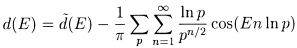where the first sum includes all primes p, and the mean density of states is given byor using Stirling's formula, as E tends to infinity, by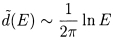."

E.B. Bogomolny and J.P. Keating, "Random matrix theory and the Riemann zeros I: three- and four-point correlations", Nonlinearity 8 (1995) 1115–1131

 H.M. Edwards, Riemann's Zeta Function, Academic Press, New York, London, 1974.

 D. Zagier, "The first 50 million prime numbers", Mathematical Intelligencer 0 (1977), 7–19.

 J. Van de Lune, H.J.J. te Riele, and D.T. Winter, "On the zeros of the Riemann zeta function in the critical strip. IV", Math. Comp. 46 (1986), 667–681.

the more elegant approach
number theory and physics archive      prime numbers: FAQ and resources
mystery      new      search      home